#### 期刊菜单

The Controlling of Local Quantum Uncertainty of Two Atoms in the Composite Cavity Optomechanical Systems and Phase-Damping Environments
DOI: 10.12677/MP.2022.123004, PDF, HTML, XML, 下载: 75  浏览: 107  国家自然科学基金支持

Abstract: Local quantum uncertainty (LQU) is one of the important measures for characterizing quantum correlations and it plays an integral role in quantum information processing. In this paper, we have studied the effect of dephasing parameters on LQU in the composite cavity optomechanical system and the controlling of various parameters in the system on LQU of two atoms. The results show that as the dephasing parameter increases, the LQU of two atoms decays with a greater decay rate. But, this decay rate is much slower than that of the concurrence, it indicates that LQU is more suitable for characterizing quantum correlations in composite cavity optomechanical systems than concurrence. By increasing the atom-cavityfield (with vibrating mirror) coupling and the vibrating mirror-cavityfield coupling, as well as both increasing the atom-cavityfield (J-C model) coupling and the atom-cavityfield (with vibrating mirror) coupling, the quantum correlation of two atoms can achieve the enhancement in the composite cavity optomechanical system. Furthermore, adjusting the atom-cavityfield (with vibrating mirror) coupling and the mirror-cavityfield coupling can effectively control the period of LQU evolution with time.

1. 引言

2. 系统模型Figure 1. The schematic diagram of the composite cavity optomechanical system

$\begin{array}{c}{H}_{I}=\hslash {g}_{1}\left({a}_{1}{\sigma }_{+}^{1}+{a}_{1}^{†}{\sigma }_{-}^{1}\right)+\frac{\hslash G{g}_{2}}{{\omega }_{m}}\left({a}_{2}^{†}{b}_{2}{\sigma }_{-}^{2}+{a}_{2}{b}_{2}^{†}{\sigma }_{+}^{2}\right)\\ \text{\hspace{0.17em}}\text{ }\text{ }-\frac{\hslash {G}^{2}}{{\omega }_{m}}{\left({a}_{2}^{†}{a}_{2}\right)}^{2}-\frac{\hslash {g}_{2}^{2}}{{\omega }_{m}}\left({a}_{2}^{†}{a}_{2}{\sigma }_{2}^{z}+{\sigma }_{+}^{2}{\sigma }_{-}^{2}\right)\end{array}$ (1)

${H}_{I}={g}_{1}\left({a}_{1}{\sigma }_{+}^{1}+{a}_{1}^{†}{\sigma }_{-}^{1}\right)+{k}_{1}\left({a}_{2}^{†}{b}_{2}{\sigma }_{-}^{2}+{a}_{2}{b}_{2}^{†}{\sigma }_{+}^{2}\right)-{k}_{2}{\left({a}_{2}^{†}{a}_{2}\right)}^{2}-{k}_{3}\left({a}_{2}^{†}{a}_{2}{\sigma }_{z}^{2}+{\sigma }_{+}^{2}{\sigma }_{-}^{2}\right)$ (2)

${|\Psi \left(0\right)〉}_{{a}_{1}{a}_{2}}=\mathrm{cos}\alpha |{e}_{1}{g}_{2}〉+\mathrm{sin}\alpha |{g}_{1}{e}_{2}〉$ (3)

$|\Psi \left(0\right)〉=\mathrm{cos}\alpha |{e}_{1}{g}_{2}〉|{0}_{m}{0}_{f1}{1}_{f2}〉+\mathrm{sin}\alpha |{g}_{1}{e}_{2}〉|{0}_{m}{0}_{f1}{1}_{f2}〉$ (4)

$\begin{array}{c}|\Psi \left(t\right)〉=A\left(t\right)|{e}_{1}{g}_{2}{0}_{m}{0}_{f1}{1}_{f2}〉+B\left(t\right)|{g}_{1}{e}_{2}{0}_{m}{0}_{f1}{1}_{f2}〉+C\left(t\right)|{g}_{1}{g}_{2}{0}_{m}{1}_{f1}{1}_{f2}〉\\ \text{\hspace{0.17em}}\text{\hspace{0.17em}}+D\left(t\right)|{g}_{1}{e}_{2}{1}_{m}{0}_{f1}{0}_{f2}〉+E\left(t\right)|{e}_{1}{e}_{2}{1}_{m}{0}_{f1}{0}_{f2}〉\\ \text{\hspace{0.17em}}\text{\hspace{0.17em}}+F\left(t\right)|{g}_{1}{e}_{2}{1}_{m}{1}_{f1}{0}_{f2}〉+S\left(t\right)|{g}_{1}{g}_{2}{0}_{m}{0}_{f1}{1}_{f2}〉\end{array}$ (5)

$i\hslash \frac{\text{d}}{\text{d}t}|\Psi \left(t\right)〉=H|\Psi \left(t\right)〉$ (6)

$\begin{array}{l}{M}_{1}\left(0\right)=\mathrm{cos}\alpha \\ {M}_{2}\left(0\right)=\mathrm{sin}\alpha \\ {M}_{3}\left(0\right)={M}_{4}\left(0\right)={M}_{5}\left(0\right)={M}_{6}\left(0\right)={M}_{7}\left(0\right)=0\end{array}$ (7)

$\begin{array}{l}A\left(t\right)=\frac{a\cdot \mathrm{cos}\left({g}_{1}t\right)}{R}\left[R\mathrm{cos}\frac{Rt}{2}+i\mathrm{sin}\frac{Rt}{2}\left({k}_{2}-2{k}_{3}\right)\right]\mathrm{exp}\left(\frac{i}{2}{k}_{2}t\right)\\ B\left(t\right)=b\cdot \mathrm{exp}\left[i\left({k}_{2}+2{k}_{3}\right)t\right]\\ C\left(t\right)=-\frac{ia\cdot \mathrm{sin}\left({g}_{1}t\right)}{R}\left[R\mathrm{cos}\frac{Rt}{2}+i\mathrm{sin}\frac{Rt}{2}\left({k}_{2}-2{k}_{3}\right)\right]\mathrm{exp}\left(\frac{i}{2}{k}_{2}t\right)\\ D\left(t\right)=-\frac{2ia\cdot \mathrm{cos}\left({g}_{1}t\right)\mathrm{sin}\frac{Rt}{2}}{R}\mathrm{exp}\left(\frac{i}{2}{k}_{2}t\right)\\ E\left(t\right)=-\frac{2a\cdot \mathrm{sin}\left({g}_{1}t\right)\mathrm{sin}\frac{Rt}{2}}{R}\mathrm{exp}\left(\frac{i}{2}{k}_{2}t\right)\\ F\left(t\right)=S\left(t\right)=0\end{array}$ (8)

$\begin{array}{c}{\rho }_{{a}_{1}{a}_{2}}\left(t\right)=A\left(t\right)A{\left(t\right)}^{*}|{e}_{1}{g}_{2}〉〈{e}_{1}{g}_{2}|+A\left(t\right)B{\left(t\right)}^{*}|{e}_{1}{g}_{2}〉〈{e}_{1}{g}_{2}|+B\left(t\right)A{\left(t\right)}^{*}|{e}_{1}{g}_{2}〉〈{e}_{1}{g}_{2}|\\ \text{\hspace{0.17em}}\text{\hspace{0.17em}}+B\left(t\right)B{\left(t\right)}^{*}|{g}_{1}{e}_{2}〉〈{g}_{1}{e}_{2}|+C\left(t\right)C{\left(t\right)}^{*}|{g}_{1}{g}_{2}〉〈{g}_{1}{g}_{2}|\\ \text{\hspace{0.17em}}\text{\hspace{0.17em}}+E\left(t\right)E{\left(t\right)}^{*}|{e}_{1}{e}_{2}〉〈{e}_{1}{e}_{2}|+F\left(t\right)F{\left(t\right)}^{*}|{g}_{1}{e}_{2}〉〈{g}_{1}{e}_{2}|\end{array}$ (9)

${\rho }_{{a}_{1}{a}_{2}}\left(t\right)=\left(\begin{array}{cccc}{|E\left(t\right)|}^{2}& 0& 0& 0\\ 0& {|A\left(t\right)|}^{2}& A\left(t\right)B{\left(t\right)}^{*}& 0\\ 0& B\left(t\right)A{\left(t\right)}^{*}& {|B\left(t\right)|}^{2}+{|F\left(t\right)|}^{2}& 0\\ 0& 0& 0& {|C\left(t\right)|}^{2}\end{array}\right)$ (10)

$\epsilon \left(\rho \right)=\underset{i,j}{\sum }\left({K}_{i}\otimes {K}_{j}\right)\rho {\left({K}_{i}\otimes {K}_{j}\right)}^{†}$ (11)

${K}_{0}=\left(\begin{array}{cc}1& 0\\ 0& \sqrt{1-\lambda }\end{array}\right),{K}_{1}=\left(\begin{array}{cc}0& 0\\ 0& \sqrt{\lambda }\end{array}\right)$ (12)

3. 局部量子不确定性与量子纠缠

$\mathcal{U}\left({\rho }_{AB}\right)=\underset{{K}_{A}}{\mathrm{min}}I\left({\rho }_{AB},{K}_{A}\otimes 1{\text{l}}_{B}\right)$ (13)

$\mathcal{U}\left(\rho \right)=1-{\lambda }_{\mathrm{max}}\left\{W\right\}$ (14)

${w}_{ij}=Tr\left\{\sqrt{\rho }\left({\sigma }_{i}\otimes 1{\text{l}}_{B}\right)\sqrt{\rho }\left({\sigma }_{j}\otimes 1{\text{l}}_{B}\right)\right\}$ (15)

$\rho =\left(\begin{array}{cc}\begin{array}{cc}{\rho }_{11}& 0\\ 0& {\rho }_{22}\end{array}& \begin{array}{cc}0& {\rho }_{14}\\ {\rho }_{23}& 0\end{array}\\ \begin{array}{cc}0& {\rho }_{32}\\ {\rho }_{41}& 0\end{array}& \begin{array}{cc}{\rho }_{33}& 0\\ 0& {\rho }_{44}\end{array}\end{array}\right)$ (16)

$\rho =\frac{1}{4}\underset{\alpha ,\beta }{\sum }{T}_{\alpha \beta }{\sigma }_{\alpha }\otimes {\sigma }_{\beta }$ (17)

$\begin{array}{l}{w}_{11}=\left(\sqrt{{\lambda }_{1}}+\sqrt{{\lambda }_{4}}\right)\left(\sqrt{{\lambda }_{2}}+\sqrt{{\lambda }_{3}}\right)+\frac{1}{4}\frac{\left({T}_{11}^{2}-{T}_{22}^{2}\right)+\left({T}_{12}^{2}-{T}_{21}^{2}\right)+\left({T}_{03}^{2}-{T}_{30}^{2}\right)}{\left(\sqrt{{\lambda }_{1}}+\sqrt{{\lambda }_{4}}\right)\left(\sqrt{{\lambda }_{2}}+\sqrt{{\lambda }_{3}}\right)}\\ {w}_{22}=\left(\sqrt{{\lambda }_{1}}+\sqrt{{\lambda }_{4}}\right)\left(\sqrt{{\lambda }_{2}}+\sqrt{{\lambda }_{3}}\right)+\frac{1}{4}\frac{\left({T}_{22}^{2}-{T}_{11}^{2}\right)+\left({T}_{21}^{2}-{T}_{12}^{2}\right)+\left({T}_{30}^{2}-{T}_{03}^{2}\right)}{\left(\sqrt{{\lambda }_{1}}+\sqrt{{\lambda }_{4}}\right)\left(\sqrt{{\lambda }_{2}}+\sqrt{{\lambda }_{3}}\right)}\\ {w}_{33}=\frac{1}{2}\left[{\left(\sqrt{{\lambda }_{1}}+\sqrt{{\lambda }_{4}}\right)}^{2}+{\left(\sqrt{{\lambda }_{2}}+\sqrt{{\lambda }_{3}}\right)}^{2}\right]+\frac{1}{8}\left[\frac{{\left({T}_{30}+{T}_{03}\right)}^{2}-{\left({T}_{11}-{T}_{22}\right)}^{2}-{\left({T}_{12}+{T}_{21}\right)}^{2}}{{\left(\sqrt{{\lambda }_{1}}+\sqrt{{\lambda }_{4}}\right)}^{2}}\right]\\ \text{\hspace{0.17em}}\text{\hspace{0.17em}}\text{\hspace{0.17em}}\text{\hspace{0.17em}}\text{\hspace{0.17em}}\text{\hspace{0.17em}}\text{\hspace{0.17em}}\text{\hspace{0.17em}}+\frac{1}{8}\left[\frac{{\left({T}_{03}-{T}_{30}\right)}^{2}-{\left({T}_{11}+{T}_{22}\right)}^{2}-{\left({T}_{12}-{T}_{21}\right)}^{2}}{{\left(\sqrt{{\lambda }_{2}}+\sqrt{{\lambda }_{3}}\right)}^{2}}\right]\\ {w}_{12}={w}_{21}=\frac{1}{2}\frac{{T}_{11}{T}_{21}+{T}_{22}{T}_{12}}{\left(\sqrt{{\lambda }_{1}}+\sqrt{{\lambda }_{4}}\right)\left(\sqrt{{\lambda }_{2}}+\sqrt{{\lambda }_{3}}\right)}\\ {w}_{13}={w}_{31}={w}_{23}={w}_{32}=0\end{array}$ (18)

$C\left(t\right)=2\mathrm{max}\left\{0,|{\rho }_{23}|-\sqrt{{\rho }_{11}{\rho }_{44}},|{\rho }_{14}|-\sqrt{{\rho }_{22}{\rho }_{33}}\right\}$ (19)

4. 数值结果与分析

4.1. 复合腔光力学系统的相位阻尼参数对LQU的影响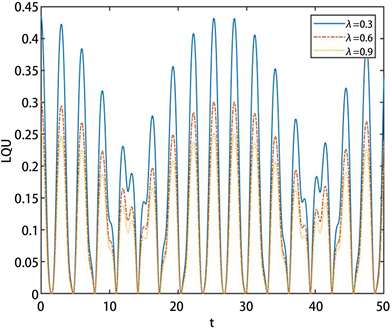Figure 2. The effect of dephasing parameters on LQU of two atomsin the composite cavity optomechanical system, where $\alpha =\pi /4$, ${g}_{1}={g}_{2}=1$, and the dephasing parameters $\lambda =0.3,0.6,0.9$ correspond to the solid, dotted and dashed lines respectively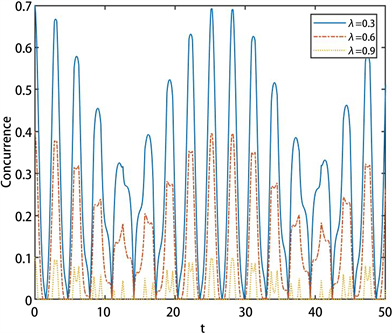Figure 3. The effect of dephasing parameters on Concurrence of two atoms in the composite cavity optomechanical system, where $\alpha =\pi /4$, ${g}_{1}={g}_{2}=1$, the dephasing parameters $\lambda =0.3,0.6,0.9$ correspond to the solid, dotted and dashed lines respectively

4.2. 相位阻尼噪声环境下复合腔光力学系统的量子关联调控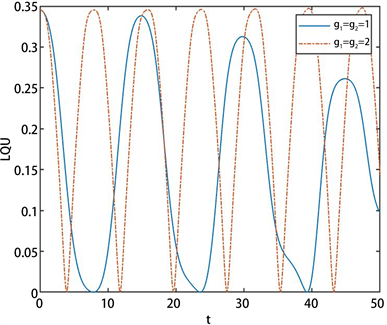Figure 4. The controlling of the atom-cavityfield (J-C model) coupling ${g}_{1}$ and the atom-cavityfield (with vibrating mirror) coupling ${g}_{2}$ on the LQU of two atoms in the composite cavity optomechanical system, where $\alpha =\pi /6$, $\lambda =0.3$, ${g}_{1}={g}_{2}=1$ and ${g}_{1}={g}_{2}=2$ correspond to the solid and dotted lines respectively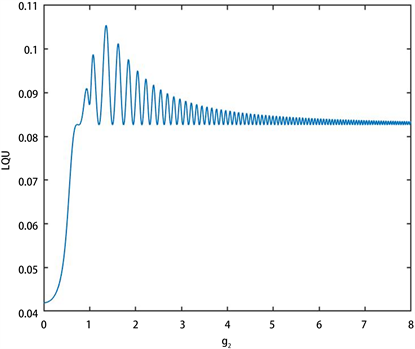Figure 5. The controlling of the atom-cavityfield (with vibrating mirror) coupling ${g}_{2}$ on the LQU of two atoms in the composite cavity optomechanical system, where $t=4.1$, $\lambda =0.5$, $\alpha =\pi /12$, ${g}_{1}=1$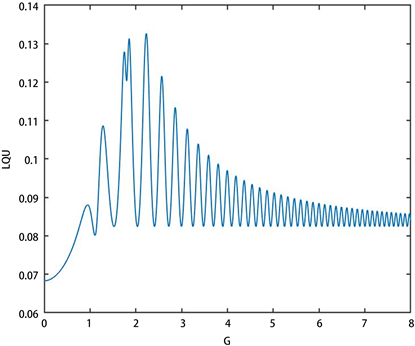Figure 6. The controlling of the vibrating mirror-cavityfield coupling G on the LQU of two atoms in the composite cavity optomechanical system, where $t=4.1$, $\lambda =0.5$, $\alpha =\pi /12$, ${g}_{1}=1$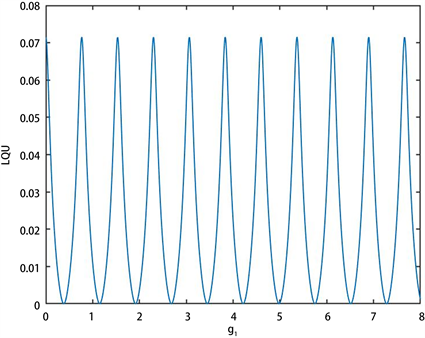Figure 7. The controlling of the atom-cavityfield (J-C model) coupling ${g}_{1}$ on the LQU of two atoms in the composite cavity optomechanical system, where $t=4.1$, $\lambda =0.5$, $\alpha =\pi /12$, ${g}_{2}=1$

5. 结论

  Bellac, M.L. (2006) A Short Introduction to Quantum Information and Quantum Computation. Cambridge University Press, Cambridge.  Girolami, D., Tufarelli, T. and Adesso, G. (2013) Characterizing Nonclassical Correlations via Local Quantum Uncertainty. Physical Review Letters, 110, Article ID: 240402. https://doi.org/10.1103/PhysRevLett.110.240402  Slaoui, A., Bakmou, L., Daoud, M. and Laamara, R.A. (2019) A Comparative Study of Local Quantum Fisher Information and Local Quantum Uncertainty in Heisenberg XY Model. Physics Letters A, 383, 2241-2247. https://doi.org/10.1016/j.physleta.2019.04.040  Ali, M. (2020) Local Quantum Uncertainty for Multipartite Quantum Systems. The European Physical Journal D, 74, 1-6. https://doi.org/10.1140/epjd/e2020-10091-x  曹蕾. 多体系统中基于局域量子不确定性的量子关联特性的研究[D]: [硕士学位论文]. 南京: 南京邮电大学, 2016.  Coulamy, I.B., Warnes, J.H., Sarandy, M.S. and Saguia, A. (2016) Scaling of the Local Quantum Uncer-tainty at Quantum Phase Transitions. Physics Letters A, 380, 1724-1728. https://doi.org/10.1016/j.physleta.2016.03.026  Carrijo, T.M., Avelar, A.T. and Celeri, L.C. (2015) Quantum Uncertainty in Critical Systems with Three Spins Interaction. Journal of Physics B Atomic Molecular and Optical Physics: An Institute of Physics Journal, 48, Article ID: 125501. https://doi.org/10.1088/0953-4075/48/12/125501  He, Z., Yao, C., Wang, Q. and Zou, J. (2014) Measuring Non-Markovianity Based on Local Quantum Uncertainty. Physical Review A, 90, Article ID: 042101. https://doi.org/10.1103/PhysRevA.90.042101  王铁. 腔光力学系统中光学响应及量子纠缠研究[D]: [硕士学位论文]. 延吉: 延边大学, 2019.  Liao, Q.H., Yuan, L., Fu, Y.C. and Zhou, N.R. (2019) Properties of Entanglement between the JC Model and Atom-Cavity-Optomechanical System. International Journal of Theoretical Physics, 58, 2641-2653. https://doi.org/10.1007/s10773-019-04152-1  Wigner, E.P. and Yanase, M.M. (1963) Information Contents of Distributions. Proceedings of the National Academy of Sciences of the United States of America, 49, 910. https://doi.org/10.1073/pnas.49.6.910